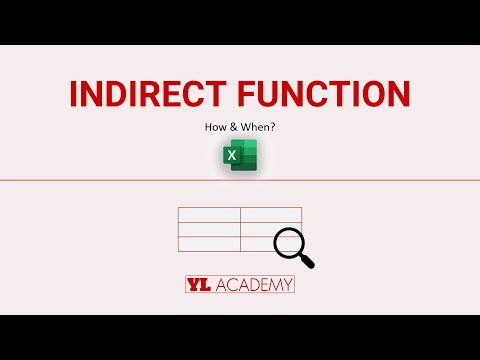What is an INDIRECT Function in Excel? - KING OF EXCELKINGEXCEL.INFO ( KING OF EXCEL ) Welcome KINGEXCEL.INFO - Nothing Is Unable ... About Excel Tricks, Learning VBA Programming, Dedicated Software, Accounting, Living Skills ...

## What is an INDIRECT Function in Excel?

The INDIRECT Function in Excel helps you to return a reference to a range. Even when you insert Rows or Columns in a Worksheet the reference you created won’t change. This will help you to create a reference from letters and numbers in other cells.## How INDIRECT Function in Excel Works with an Example

The INDIRECT function is useful when you want to return a value, based on a text string. Example: You’ll be able to see the list of food items and their Consumption by Countries such as USA, China and Japan. The quantity is in Million i.e. 1 Million=10,00,000

Problem: You need to find out:
• An independent Cell Value
• SUM consumption of the Food items of USA
• MAX Value of Food Consumption of Japan
• MIN Value of Food Consumption of CHINA
Question: How will you find the Values?

### INDIRECT Formula to find an independent Cell Value

1. In the Cell F3, Type =INDIRECT(for ref_text give the reference of the above Cell, Select F2) i.e. =INDIRECT(F2)
2. You get #REF! (This is because there was no Cell Value assigned to the Cell F2)
3. Select C5 and Enter C5 as the Cell Value at the place of F2. You get Value 150
(The Error text disappears and you get the Value 150 i.e. the Value of Silk Consumption in China)

### INDIRECT Formula to find a Sum consumption

• Select the range of Cells for the Columns USA, CHINA and JAPAN
• Provide a Table Name to USA, CHINA and JAPAN respectively in the NAME BOX
• Type the name USA in the Cell F2
• In the Cell F3Type =SUM(INDIRECT(F2))
Note 1: Don’t forget to close the Bracket for SUM & INDIRECT both
Note 2: If you don’t Select the Table Range and Name your Table then Typing USA won’t give the SUM in Excel. You must Give your Table a name in the NAME BOX
• Press Enter. You’ll get the Value 1250
(You can Cross-Check the Total by Calculating the Total Values of USA. The Answer remains same)
Note: You can change the Country Name to China and Japan Respectively to get the Sum of the Country

### INDIRECT Formula to find MAX Value

• In the Cell F2Type JAPAN
• In the Cell F3Type =MAX(=INDIRECT(F2))
• Press Enter. You’ll get the Value 450
Note: If you don’t Select the Table Range and Name your Table then Typing USA won’t give the SUM in Excel. You must give your Table a name in the NAME BOX
(You can Cross Check the MAX Value by Checking out the Column of Japan. The Answer remains same)

### INDIRECT Formula to find MIN Value

• In the Cell F2Type CHINA
• In the Cell F3Type =MIN(=INDIRECT(F2))
• Press Enter. You’ll get the Value 150
Note: If you don’t Select the Table Range and Name your Table then Typing USA won’t give the SUM in Excel. You must give your Table a name in the NAME BOX
(You can Cross-Check the MIN Value by Checking out the Column of Japan. The Answer remains same)
‘Excel reference cell in another sheet dynamically’?
Excel INDIRECT function – syntax and basic uses
As you understand from the Word, ‘Excel INDIRECT’, it helps to indirectly reference cells, ranges, other sheets or workbooks.
In other words, The INDIRECT function in Excel makes it easy for you to create an Excel dynamic cell reference or range reference. You do not have to do the “hard-coding” of the references into your Excel formulas.
This results in the change of a Cell Reference within a formula without changing the formula itself. More importantly, these indirect references in the INDIRECT Formula in Excel do not change when a new row or column is added or inserted in the worksheet. Also, the Cell Reference remains the same when you delete the existing Rows and Columns. When some formulas get extended you can use wrap text in Excel
If you wish to Write an INDIRECT Function in Excel, you need to understand the Basics of Function Arguments.

## What if your Indirect formula not working?

Situation: You’re using the indirect formula in Excel to pull data of other tabs.
These tab names are compiled and placed on a tab through VBA code (this is a clear cut and paste)
Problem: The Indirect formula is unable to calculate because what I am assuming is the format of the data which is present in column B
It is observed that: Whenever you type over the data (which is exactly the same) this excel formula works.
Solution: Before you overwrite, you need to check:
• If there are unseen Whitespace Characters in the Cell
• If something is Misspelled
If this doesn’t work then, you can try this:
=IF(ISERROR(INDIRECT(TRIM(\$B2)&”!U2″)),””,INDIRECT(TRIM(\$B2)&”!U2″))
#evba #etipfree #kingexcel
📤You download App EVBA.info installed directly on the latest phone here : https://www.evba.info/p/app-evbainfo-setting-for-your-phone.html?m=1